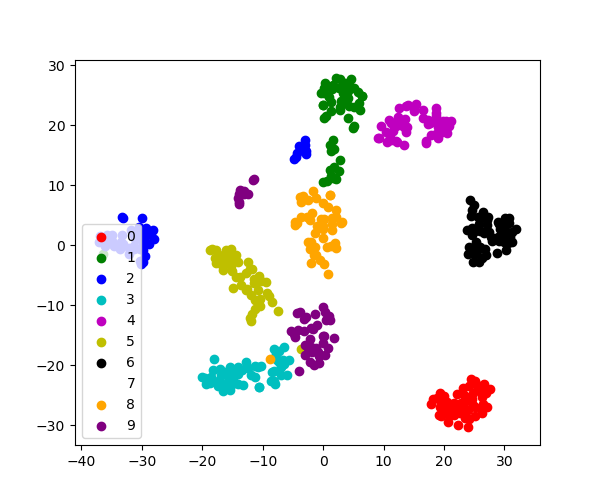# 3.6.10.5. tSNE to visualize digits¶

Here we use `sklearn.manifold.TSNE` to visualize the digits datasets. Indeed, the digits are vectors in a 8*8 = 64 dimensional space. We want to project them in 2D for visualization. tSNE is often a good solution, as it groups and separates data points based on their local relationship.

```from sklearn import datasets
# Take the first 500 data points: it's hard to see 1500 points
X = digits.data[:500]
y = digits.target[:500]
```

Fit and transform with a TSNE

```from sklearn.manifold import TSNE
tsne = TSNE(n_components=2, random_state=0)
```

Project the data in 2D

```X_2d = tsne.fit_transform(X)
```

Visualize the data

```target_ids = range(len(digits.target_names))

from matplotlib import pyplot as plt
plt.figure(figsize=(6, 5))
colors = 'r', 'g', 'b', 'c', 'm', 'y', 'k', 'w', 'orange', 'purple'
for i, c, label in zip(target_ids, colors, digits.target_names):
plt.scatter(X_2d[y == i, 0], X_2d[y == i, 1], c=c, label=label)
plt.legend()
plt.show()
```Total running time of the script: ( 0 minutes 1.335 seconds)

Gallery generated by Sphinx-Gallery